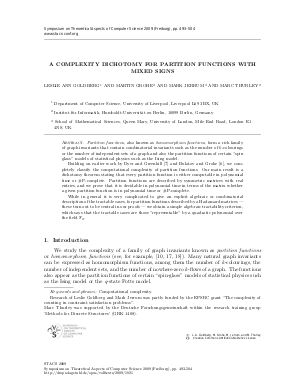Document# A Complexity Dichotomy for Partition Functions with Mixed Signs

### Authors Leslie Ann Goldberg, Martin Grohe, Mark Jerrum, Marc Thurley## File

LIPIcs.STACS.2009.1821.pdf
• Filesize: 195 kB
• 12 pages

## Cite As

Leslie Ann Goldberg, Martin Grohe, Mark Jerrum, and Marc Thurley. A Complexity Dichotomy for Partition Functions with Mixed Signs. In 26th International Symposium on Theoretical Aspects of Computer Science. Leibniz International Proceedings in Informatics (LIPIcs), Volume 3, pp. 493-504, Schloss Dagstuhl - Leibniz-Zentrum für Informatik (2009)
https://doi.org/10.4230/LIPIcs.STACS.2009.1821

## Abstract

\emph{Partition functions}, also known as \emph{homomorphism functions}, form a rich family of graph invariants that contain combinatorial invariants such as the number of $k$-colourings or the number of independent sets of a graph and also the partition functions of certain spin glass'' models of statistical physics such as the Ising model. Building on earlier work by Dyer and Greenhill (2000) and Bulatov and Grohe (2005), we completely classify the computational complexity of partition functions. Our main result is a dichotomy theorem stating that every partition function is either computable in polynomial time or \#P-complete. Partition functions are described by symmetric matrices with real entries, and we prove that it is decidable in polynomial time in terms of the matrix whether a given partition function is in polynomial time or \#P-complete. While in general it is very complicated to give an explicit algebraic or combinatorial description of the tractable cases, for partition functions described by a Hadamard matrices --- these turn out to be central in our proofs --- we obtain a simple algebraic tractability criterion, which says that the tractable cases are those representable'' by a quadratic polynomial over the field $\ensuremath{\mathbb{F}_2}$.
##### Keywords
• Computational complexity

## Metrics

• Access Statistics
• Total Accesses (updated on a weekly basis)
0